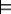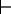### 5.2.2 Proofs

So far, we have specified what an answer is, but not how it can be computed. The definition ofspecifies what propositions should be logical consequences of a knowledge base but not how to compute them. The problem of deduction is to determine if some proposition is a logical consequence of a knowledge base. Deduction is a specific form of inference.

A proof is a mechanically derivable demonstration that a proposition logically follows from a knowledge base. A theorem is a provable proposition. A proof procedure is a - possibly non-deterministic - algorithm for deriving consequences of a knowledge base. See the box for a description of non-deterministic choice.

Given a proof procedure, KBg means g can be proved or derived from knowledge base KB.

A proof procedure's quality can be judged by whether it computes what it is meant to compute.

A proof procedure is sound with respect to a semantics if everything that can be derived from a knowledge base is a logical consequence of the knowledge base. That is, if KBg, then KBg.

A proof procedure is complete with respect to a semantics if there is a proof of each logical consequence of the knowledge base. That is, if KBg, then KBg.

We present two ways to construct proofs for propositional definite clauses: a bottom-up procedure and a top-down procedure.